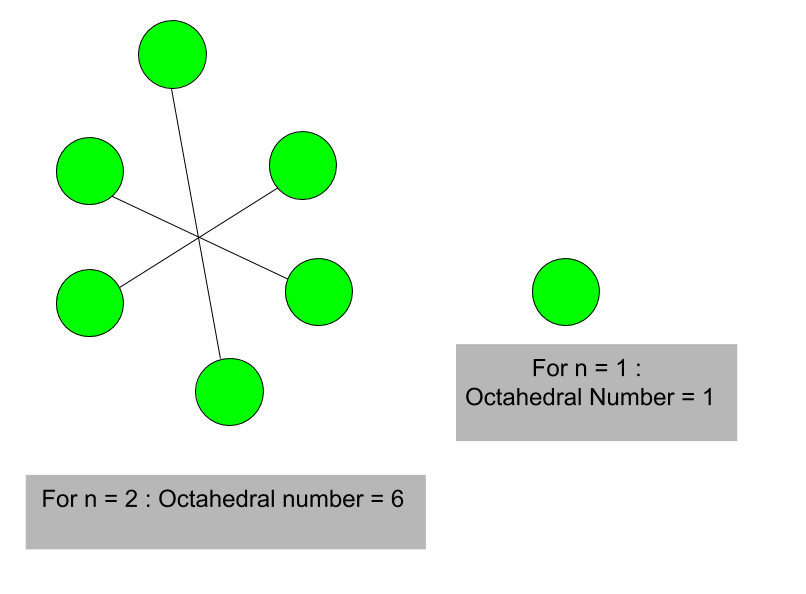# Octahedral Number

Given a number n, the task is to find n-th octahedral number.
A octahedral number belongs to a figurate number and it is the number of spheres in an octahedron built from closely packed spheres. First few octahedral numbers (where n = 0, 1, 2, 3…….) are : 0, 1, 6, 19 and so on.

Examples :

Input : 4
Output : 44

Input : 8
Output : 344Formula for nth octahedral number:

`n * (2n2+1) / 3`

## C++

 `// C++ program to find nth ` `// octahedral number ` `#include ` `using` `namespace` `std; ` ` `  `// Function to find ` `// octahedral number ` `int` `octahedral_num(``int` `n) ` `{ ` `    ``// Formula to calculate nth ` `    ``// octahedral number ` `    ``return` `n * (2 * n * n + 1) / 3; ` `} ` ` `  `// Drivers code ` `int` `main() ` `{ ` `    ``int` `n = 5; ` ` `  `    ``// print result ` `    ``cout << n << ``"th Octahedral number: "``; ` `    ``cout << octahedral_num(n); ` `    ``return` `0; ` `} `

## Java

 `// Java program to find nth octahedral ` `// number ` `import` `java.io.*; ` ` `  `class` `GFG { ` ` `  `    ``// Function to find octahedral number ` `    ``static` `int` `octahedral_num(``int` `n) ` `    ``{ ` ` `  `        ``// Formula to calculate nth ` `        ``// octahedral number ` `        ``// and return it into main function. ` `        ``return` `n * (``2` `* n * n + ``1``) / ``3``; ` `    ``} ` ` `  `    ``// Driver Code ` `    ``public` `static` `void` `main(String[] args) ` `    ``{ ` `        ``int` `n = ``5``; ` `        ``// print result ` `        ``System.out.print(n + ``"th Octahedral"` `                         ``+ ``" number: "``); ` `        ``System.out.println(octahedral_num(n)); ` `    ``} ` `} `

## Python3

 `# Python 3 program to find nth ` `# octahedral number ` ` `  `# Function to find ` `# octahedral number ` `def` `octahedral_num(n) : ` `     `  `    ``# Formula to calculate nth ` `    ``# octahedral number ` `    ``return` `n ``*` `(``2` `*` `n ``*` `n ``+` `1``) ``/``/` `3` ` `  `# Driver Code ` `if` `__name__ ``=``=` `'__main__'` `: ` `         `  `    ``n ``=` `5` `    ``print``(n,``"th Octahedral number: "` `                ``, octahedral_num(n)) ` ` `  `# This code is contributed ajit. `

## C#

 `// C# program to find nth  ` `// Octahedral number ` `using` `System; ` ` `  `class` `GFG ` `{ ` `     `  `    ``// Function to find  ` `    ``// octahedral number ` `    ``static` `int` `octahedral_num(``int` `n) ` `    ``{ ` ` `  `        ``// Formula to calculate  ` `        ``// nth octahedral number ` `        ``// and return it into  ` `        ``// main function. ` `        ``return` `n * (2 * n *  ` `                    ``n + 1) / 3; ` `    ``} ` ` `  `    ``// Driver Code ` `    ``static` `public` `void` `Main () ` `    ``{ ` `        ``int` `n = 5; ` `         `  `        ``// print result ` `        ``Console.Write(n + ``"th Octahedral"` `                        ``+ ``" number: "``); ` `        ``Console.WriteLine(octahedral_num(n)); ` `    ``} ` `} ` ` `  `// This code is Contributed by m_kit `

## PHP

 `  `

Output :

```5th Octahedral number: 85
```

My Personal Notes arrow_drop_upIf you like GeeksforGeeks and would like to contribute, you can also write an article using contribute.geeksforgeeks.org or mail your article to contribute@geeksforgeeks.org. See your article appearing on the GeeksforGeeks main page and help other Geeks.

Please Improve this article if you find anything incorrect by clicking on the "Improve Article" button below.

Improved By : jit_t

Article Tags :
Practice Tags :

Be the First to upvote.

Please write to us at contribute@geeksforgeeks.org to report any issue with the above content.